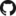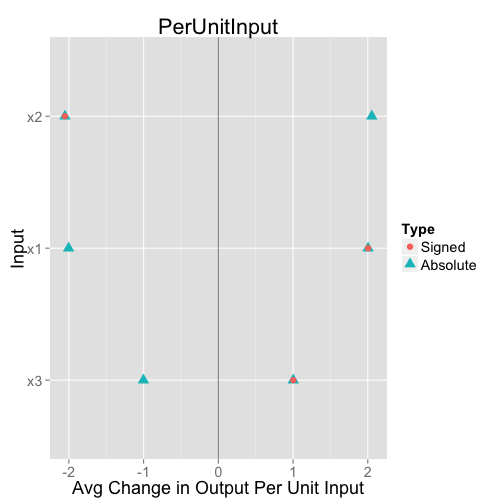# PredcompsHome

PDF Manual

Per Unit Input (original APC)

Impact (in units of output)

## Examples

Examples Overview

A fake logistic regression example predicting wine sales

A linear model with interactions

Loan defaults example

## More

As compared with Gelman & Pardoe 2007

Pairs & Weights

## APCs

### Predictive Comparisons Generalize Regression Coefficients

At the heart of this package is the idea of a predictive comparison: We vary the input of interest holding the other inputs constant, and look at the differences in predicted values. Let $$u$$ represent the input of interest and $$v$$ the (vector of) other inputs. Let $$f$$ be a functinon making predictions, so

$\hat{y} = f(u,v)$

Our $$f$$ could come from any predictive model. If we have a statistical model, then probably we would choose

$f(u,v) = \mathcal{E}[y \mid u, v, \theta]$

(where $$\theta$$ are the parameters of the model). But we need not have a statistical model at all. For example, the prediction function $$f$$ could come from a random forest, or a support vector machine.

Given the function $$f$$ and a choice of $$u_1$$, $$u_2$$, and $$v$$, we can compute

$\delta_{u_1 \rightarrow u_2, v} = \frac{f(u_2, v) - f(u_1, v)}{u_2-u_1}$

If $$f$$ were a linear model with no interactions, the above would not depend on the particular choices of $$u_1$$, $$u_2$$, and $$v$$ and would be the regression coefficient corresponding to $$u$$. This is the formal sense in which predictive comparisons generalize regression coefficients. Since for more complicated models this varies as the inputs vary, we will take an average across a well chosen set of inputs.

### Choice of Inputs

The APC is defined as

$\frac{\mathcal{E}[\Delta_f]}{\mathcal{E}[\Delta_u]}$

where $$\Delta_f = f(u_2,v) - f(u_1,v)$$, $$\Delta_u = u_2 - u_1$$, and $$\mathcal{E}$$ is expectation under the following process:

1. sample $$v$$ from the (marginal) distribution of the corresponding inputs
2. sample $$u_1$$ and $$u_2$$ independently from the distribution of $$u$$ conditional on $$v$$

The reason for this definition is that we want to use representative values of $$v$$, and transitions in $$u$$ that are representative of what really occurs at those values of $$v$$.

The fact that we are computing the numerator and denominator separately (rather than taking an expected value of $$\delta_{u_1 \rightarrow u_2, v}$$) amounts to weighting by the size of $$(u_2 - u_1)$$. This avoids having the result excessively influenced by small changes in $$u$$.

### Estimation

The rows of our data represent samples from the joint distribution $$u$$, $$v$$, so each row amounts to a sample from $$v$$ followed by a sample $$u_1$$ conditional on $$v$$. The difficult thing is drawing another sample $$u_2$$ conditional on $$v$$. We approximate these by assigning weights to rows based on the proximity of the $$v$$ in that row to the $$v$$ in the row in question. The weights are:

$\frac{1}{\text{mahalanobisConstantTerm} + \text{(mahalanobis distance)}}$

The mahalanobis distance is a unitless version of distance that takes into account the correlation structure of $$v$$. The mahalanobisConstantTerm (which defaults to 1, but this is not always an appropriate choice) prevents all of the weight from going to the closest points. More work needs to be done in thinking about the weights.

For more details, see Gelman and Pardoe 2007 (section 4), my note explaining a small change from the weights described in the paper (renormalization) and reasons for considering only a set of the closest $$N$$ points. You can also look at my code that computes the appropriate weights.

### Absolute Version

The absolute APC (as opposed to the signed version described above) replaces $$\Delta_f = f(u_2,v) - f(u_1,v)$$ above with $$|\Delta_f| = |f(u_2,v) - f(u_1,v)|$$. For an extreme artificial example see e.g. the input $$u_8$$ in my simulated linear model with interactions, where the signed APC is roughly 0 but the absolute APC is large.

By default, I always compute and display an absolute version of the APC alongside the signed version.

### A Small Example

This is a example running APCs on a simulated linear model with independent inputs and no interactions. For more involved examples, see the examples section.

The inputs ($$x_1$$, $$x_2$$, $$x_3$$) are independent, with

$y \sim 2x_1 - 2x_2 + x_3 + \mathcal{N}(0,.1)$

First we set up the data:

n <- 200
x1 <- runif(n = n, min = 0, max = 1)
x2 <- runif(n = n, min = 0, max = 1)
x3 <- runif(n = n, min = 0, max = 10)
y <- 2 * x1 + (-2) * x2 + 1 * x3 + rnorm(n, sd = 0.1)
df <- data.frame(x1, x2, x3, y)


Then we fit a linear model:

fittedLm <- lm(y ~ ., data = df)
fittedLm

##
## Call:
## lm(formula = y ~ ., data = df)
##
## Coefficients:
## (Intercept)           x1           x2           x3
##      0.0169       2.0040      -2.0533       1.0024


We can then plot the average predictive comparisons:

library(predcomps)
apcDF <- GetPredCompsDF(fittedLm, df = df)
PlotPredCompsDF(apcDF, variant = "PerUnitInput") + theme_gray(base_size = 18)Using different shapes / colors, both the absolute and signed versions are plotted. For symmetry, the absolute version is plotted with both a positive and negative sign. Since this is a linear model with no interactions, the signed APCs match those from the fitted linear model.

The call to GetPredCompsDF returns this data frame:

apcDF

##    Input PerUnitInput.Signed PerUnitInput.Absolute Impact.Signed
## x1    x1               2.004                 2.004        0.6408
## x2    x2              -2.053                 2.053       -0.6629
## x3    x3               1.002                 1.002        3.3046
##    Impact.Absolute
## x1          0.6408
## x2          0.6629
## x3          3.3046


The columns plotted here are PerUnitInput.Signed and Apc.Absolute. The next section desceibes those columns labeled “Impact”.